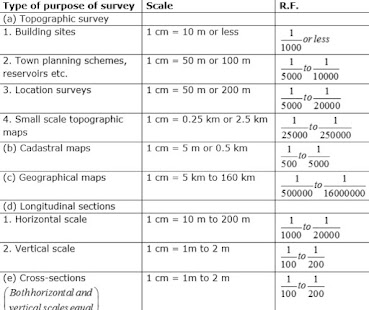# What are Scales in Civil Engineering Surveying?

Scale is a numerical factor that is used while representing the actual ground distance, elevation, or direction of a real construction site or area, in a reduced measure on paper. This visual representation of the entire area or part of the area is called a map.  Hence a map scale is defined as the ratio of the distance represented in the map to the actual distance on the ground.

A scale can be used to represent a small feature in the ground, big on the map or drawing, or vice versa.

This article discusses the R.F for scales and types of scales used in surveying.

### Types of Scales

Scale can be represented as Engineer Scale, Graphical Scale, and Representative Fraction (RF).

#### Engineer Scale

In this scale, the proportion implies every distance on the map or drawing bears the corresponding distance on the ground. For example: 1cm = 10 m.

#### Graphical Scale

A graphical scale is a line drawn on the map so that its map distance corresponds to a convenient unit of length on the ground.Scales for Different Types of Survey

### Representative Fraction (RF) for Scales in Surveying

Representative Fraction (RF) = Map Distance / Ground Distance

One inch on the map equals 24,000 inches on the ground, and one centimeter on the map equals 24,000 centimeters on the ground, then RF can be expressed as 1: 24,000.

In order to draw the map for surveying purposes, first RF is calculated based on how small or big the map needs to be. R.F is a mathematical relation between MAP and LAND.

### Types of Representative Fractions (RF) for Scales in Surveying

The three types of R.F are:
1. Reducing Scale
2. Full Scale
3. Enlarging Scale

#### 1. Reducing Scales

When the dimensions on the drawing are smaller than the actual dimensions of the object. It is represented by the scale and RF as Scale: 1 cm = 100 cm or 1: 100 and by RF = 1 / 100 (less than one)

#### 2. Full Scale

Sometimes the actual dimensions of the object will be adopted on the drawing then in that case it is represented by the scale and RF as Scale: 1 cm = 1 cm or 1:1 and by RF = 1/1 (equal to one).

#### 3. Enlarging Scale

In some cases, when the objects are very small like inside parts of a steel connection, the dimensions adopted on the drawing will be bigger than the actual dimensions of the objects then in that case it is represented by scale and RF as Scale: 10 cm = 1 cm or 10: 1 and by RF = 10 / 1 (greater than one)

Hence, R.F can be greater than 1, less than 1, or equal to 1.

### Different Types of Measuring Scales Used in Surveying

Different types of scales used in surveying are:
1. Plain Scale
2. Diagonal Scale
3. The Vernier Scale
4. Shrunk Scale

#### 1. Plain Scale

A plain scale is one on which it is possible to measure two dimensions only. This means it can measure units and lengths, diameters, miles, furlongs, etc.

#### 2. Diagonal Scale

On a diagonal scale, a maximum of three dimensions can be measured. This includes meters, decimeters, and centimeters; units, tenths, and hundreds; yards, feet, and inches.

#### 3. The Vernier Scale

Direct Vernier and Retrograde vernier are the two types of vernier scales.

If,
s= value of one smallest division of the main scale
n = number of divisions on the vernier
v = value of one smallest division of vernier

a. Direct Vernier Scale

Here vernier scale moves in the same direction of the main scale.

Then the Least Count of Direct Vernier Scale is = s/n
And they form a relationship:
nv = (n-1)s

Here, the vernier scale moves in the opposite direction of the main scale.

Then the Least Count of Retrograde Vernier Scale is = s/n
And they form a relationship:
nv = (n+1)s

#### 4. Shrunk Scale

Shrunk scale = Original Scale x shrinkage Factor

Shrinkage Factor = Shrunk Length / Actual Length